## 1.API#

### 1.1 API概述【理解】#

• 什么是API

​ API (Application Programming Interface) ：应用程序编程接口

• java中的API

​ 指的就是 JDK 中提供的各种功能的 Java类，这些类将底层的实现封装了起来，我们不需要关心这些类是如何实现的，只需要学习这些类如何使用即可，我们可以通过帮助文档来学习这些API如何使用。

### 1.2 如何使用API帮助文档【应用】#

• 打开帮助文档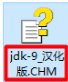• 找到索引选项卡中的输入框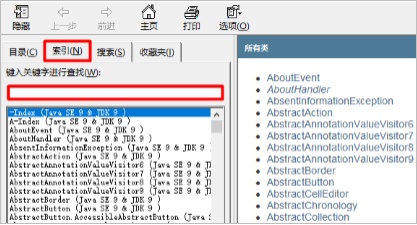• 在输入框中输入Random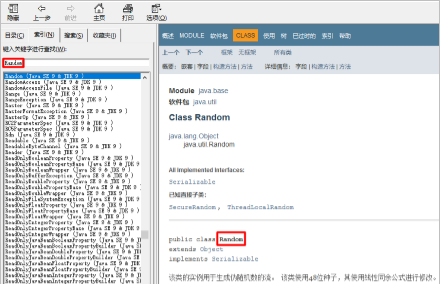• 看类在哪个包下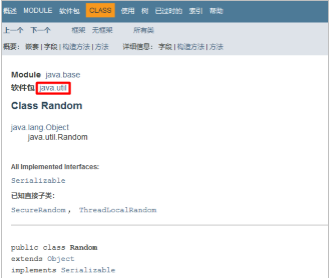• 看类的描述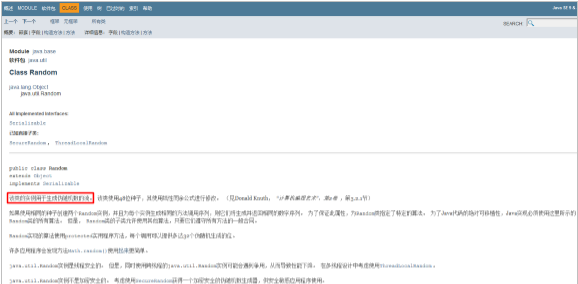• 看构造方法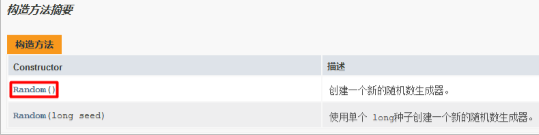• 看成员方法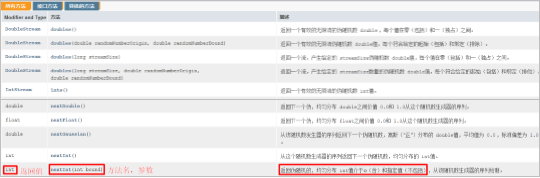## 2.常用API#

### 2.1 Math（应用）#

• 1、Math类概述

• Math 包含执行基本数字运算的方法
• 2、Math中方法的调用方式

• Math类中无构造方法，但内部的方法都是静态的，则可以通过 类名.进行调用
• 3、Math类的常用方法

方法名 方法名 说明
public static int abs(int a) 返回参数的绝对值
public static double ceil(double a) 返回大于或等于参数的最小double值，等于一个整数
public static double floor(double a) 返回小于或等于参数的最大double值，等于一个整数
public static int round(float a) 按照四舍五入返回最接近参数的int
public static int max(int a,int b) 返回两个int值中的较大值
public static int min(int a,int b) 返回两个int值中的较小值
public static double pow (double a,double b) 返回a的b次幂的值
public static double random() 返回值为double的正值，[0.0,1.0)

### 2.2 System（应用）#

• System类的常用方法

方法名 说明
public static void exit(int status) 终止当前运行的 Java 虚拟机，非零表示异常终止
public static long currentTimeMillis() 返回当前时间(以毫秒为单位)
• 示例代码

• 需求：在控制台输出1-10000，计算这段代码执行了多少毫秒
``````public class SystemDemo {
public static void main(String[] args) {
// 获取开始的时间节点
long start = System.currentTimeMillis();
for (int i = 1; i <= 10000; i++) {
System.out.println(i);
}
// 获取代码运行结束后的时间节点
long end = System.currentTimeMillis();
System.out.println("共耗时：" + (end - start) + "毫秒");
}
}
``````

### 2.3 Object类的toString方法（应用）#

• Object类概述

• Object 是类层次结构的根，每个类都可以将 Object 作为超类。所有类都直接或者间接的继承自该类，换句话说，该类所具备的方法，所有类都会有一份
• 查看方法源码的方式

• 选中方法，按下Ctrl + B
• 重写toString方法的方式

1. Alt + Insert 选择toString
1. 在类的空白区域，右键 -> Generate -> 选择toString
• toString方法的作用：

• 以良好的格式，更方便的展示对象中的属性值
• 示例代码：

``````class Student extends Object {
private String name;
private int age;

public Student() {
}

public Student(String name, int age) {
this.name = name;
this.age = age;
}

public String getName() {
return name;
}

public void setName(String name) {
this.name = name;
}

public int getAge() {
return age;
}

public void setAge(int age) {
this.age = age;
}

@Override
public String toString() {
return "Student{" +
"name='" + name + '\'' +
", age=" + age +
'}';
}
}
public class ObjectDemo {
public static void main(String[] args) {
Student s = new Student();
s.setName("林青霞");
s.setAge(30);
System.out.println(s);
System.out.println(s.toString());
}
}
``````
• 运行结果：

``````Student{name='林青霞', age=30}
Student{name='林青霞', age=30}
``````

### 2.4 Object类的equals方法（应用）#

• equals方法的作用

• 用于对象之间的比较，返回true和false的结果
• 举例：s1.equals(s2); s1和s2是两个对象
• 重写equals方法的场景

• 不希望比较对象的地址值，想要结合对象属性进行比较的时候。
• 重写equals方法的方式

1. alt + insert 选择equals() and hashCode()，IntelliJ Default，一路next，finish即可
1. 在类的空白区域，右键 -> Generate -> 选择equals() and hashCode()，后面的同上。
• 示例代码：

``````class Student {
private String name;
private int age;

public Student() {
}

public Student(String name, int age) {
this.name = name;
this.age = age;
}

public String getName() {
return name;
}

public void setName(String name) {
this.name = name;
}

public int getAge() {
return age;
}

public void setAge(int age) {
this.age = age;
}

@Override
public boolean equals(Object o) {
//this -- s1
//o -- s2
if (this == o) return true;
if (o == null || getClass() != o.getClass()) return false;

Student student = (Student) o; //student -- s2

if (age != student.age) return false;
return name != null ? name.equals(student.name) : student.name == null;
}
}
public class ObjectDemo {
public static void main(String[] args) {
Student s1 = new Student();
s1.setName("林青霞");
s1.setAge(30);

Student s2 = new Student();
s2.setName("林青霞");
s2.setAge(30);

//需求：比较两个对象的内容是否相同
System.out.println(s1.equals(s2));
}
}
``````
• 面试题

``````// 看程序,分析结果
String s = “abc”;
StringBuilder sb = new StringBuilder(“abc”);
s.equals(sb);
sb.equals(s);

public class InterviewTest {
public static void main(String[] args) {
String s1 = "abc";
StringBuilder sb = new StringBuilder("abc");
//1.此时调用的是String类中的equals方法.
//保证参数也是字符串,否则不会比较属性值而直接返回false
//System.out.println(s1.equals(sb)); // false

//StringBuilder类中是没有重写equals方法,用的就是Object类中的.
System.out.println(sb.equals(s1)); // false
}
}
``````

### 2.5 Objects (应用)#

• 常用方法

方法名 说明
public static String toString(对象) 返回参数中对象的字符串表示形式。
public static String toString(对象, 默认字符串) 返回对象的字符串表示形式。
public static Boolean isNull(对象) 判断对象是否为空
public static Boolean nonNull(对象) 判断对象是否不为空
• 示例代码

学生类

``````class Student {
private String name;
private int age;

public Student() {
}

public Student(String name, int age) {
this.name = name;
this.age = age;
}

public String getName() {
return name;
}

public void setName(String name) {
this.name = name;
}

public int getAge() {
return age;
}

public void setAge(int age) {
this.age = age;
}

@Override
public String toString() {
return "Student{" +
"name='" + name + '\'' +
", age=" + age +
'}';
}
}
``````

测试类

``````public class MyObjectsDemo {
public static void main(String[] args) {
//        public static String toString(对象): 返回参数中对象的字符串表示形式。
//        Student s = new Student("小罗同学",50);
//        String result = Objects.toString(s);
//        System.out.println(result);
//        System.out.println(s);

//        public static String toString(对象, 默认字符串): 返回对象的字符串表示形式。如果对象为空,那么返回第二个参数.
//Student s = new Student("小花同学",23);
//        Student s = null;
//        String result = Objects.toString(s, "随便写一个");
//        System.out.println(result);

//        public static Boolean isNull(对象): 判断对象是否为空
//Student s = null;
//        Student s = new Student();
//        boolean result = Objects.isNull(s);
//        System.out.println(result);

//        public static Boolean nonNull(对象): 判断对象是否不为空
//Student s = new Student();
Student s = null;
boolean result = Objects.nonNull(s);
System.out.println(result);
}
}
``````

### 2.6 BigDecimal (应用)#

• 作用

可以用来进行精确计算

• 构造方法

方法名 说明
BigDecimal(double val) 参数为double
BigDecimal(String val) 参数为String
• 常用方法

方法名 说明
public BigDecimal subtract (另一个BigDecimal对象) 减法
public BigDecimal multiply (另一个BigDecimal对象) 乘法
public BigDecimal divide (另一个BigDecimal对象) 除法
public BigDecimal divide (另一个BigDecimal对象，精确几位，舍入模式) 除法
• 总结

1. BigDecimal是用来进行精确计算的
2. 创建BigDecimal的对象，构造方法使用参数类型为字符串的。
3. 四则运算中的除法，如果除不尽请使用divide的三个参数的方法。

代码示例：

``````BigDecimal divide = bd1.divide(参与运算的对象,小数点后精确到多少位,舍入模式);
参数1 ，表示参与运算的BigDecimal 对象。
参数2 ，表示小数点后面精确到多少位
参数3 ，舍入模式
BigDecimal.ROUND_UP  进一法
BigDecimal.ROUND_FLOOR 去尾法
BigDecimal.ROUND_HALF_UP 四舍五入
``````

## 3.包装类#

### 3.1 基本类型包装类（记忆）#

• 基本类型包装类的作用

将基本数据类型封装成对象的好处在于可以在对象中定义更多的功能方法操作该数据

常用的操作之一：用于基本数据类型与字符串之间的转换

• 基本类型对应的包装类

基本数据类型 包装类
byte Byte
short Short
int Integer
long Long
float Float
double Double
char Character
boolean Boolean

### 3.2 Integer类（应用）#

• Integer类概述

包装一个对象中的原始类型 int 的值

• Integer类构造方法

方法名 说明
public Integer(int value) 根据 int 值创建 Integer 对象(过时)
public Integer(String s) 根据 String 值创建 Integer 对象(过时)
public static Integer valueOf(int i) 返回表示指定的 int 值的 Integer 实例
public static Integer valueOf(String s) 返回一个保存指定值的 Integer 对象 String
• 示例代码

``````public class IntegerDemo {
public static void main(String[] args) {
//public Integer(int value)：根据 int 值创建 Integer 对象(过时)
Integer i1 = new Integer(100);
System.out.println(i1);

//public Integer(String s)：根据 String 值创建 Integer 对象(过时)
Integer i2 = new Integer("100");
//        Integer i2 = new Integer("abc"); //NumberFormatException
System.out.println(i2);
System.out.println("--------");

//public static Integer valueOf(int i)：返回表示指定的 int 值的 Integer 实例
Integer i3 = Integer.valueOf(100);
System.out.println(i3);

//public static Integer valueOf(String s)：返回一个保存指定值的Integer对象 String
Integer i4 = Integer.valueOf("100");
System.out.println(i4);
}
}
``````

### 3.3 自动拆箱和自动装箱（理解）#

• 自动装箱

​ 把基本数据类型转换为对应的包装类类型

• 自动拆箱

​ 把包装类类型转换为对应的基本数据类型

• 示例代码

``````Integer i = 100;  // 自动装箱
i += 200;         // i = i + 200;  i + 200 自动拆箱；i = i + 200; 是自动装箱
``````

### 3.4 int和String类型的相互转换（记忆）#

• int转换为String

• 转换方式

• 方式一：直接在数字后加一个空字符串
• 方式二：通过String类静态方法valueOf()
• 示例代码

``````public class IntegerDemo {
public static void main(String[] args) {
//int --- String
int number = 100;
//方式1
String s1 = number + "";
System.out.println(s1);
//方式2
//public static String valueOf(int i)
String s2 = String.valueOf(number);
System.out.println(s2);
System.out.println("--------");
}
}
``````
• String转换为int

• 转换方式

• 方式一：先将字符串数字转成Integer，再调用valueOf()方法
• 方式二：通过Integer静态方法parseInt()进行转换
• 示例代码

``````public class IntegerDemo {
public static void main(String[] args) {
//String --- int
String s = "100";
//方式1：String --- Integer --- int
Integer i = Integer.valueOf(s);
//public int intValue()
int x = i.intValue();
System.out.println(x);
//方式2
//public static int parseInt(String s)
int y = Integer.parseInt(s);
System.out.println(y);
}
}
``````

### 3.5 字符串数据排序案例（应用）#

• 案例需求

​ 有一个字符串：“91 27 46 38 50”，请写程序实现最终输出结果是：27 38 46 50 91

• 代码实现

``````public class IntegerTest {
public static void main(String[] args) {
//定义一个字符串
String s = "91 27 46 38 50";

//把字符串中的数字数据存储到一个int类型的数组中
String[] strArray = s.split(" ");
//        for(int i=0; i<strArray.length; i++) {
//            System.out.println(strArray[i]);
//        }

//定义一个int数组，把 String[] 数组中的每一个元素存储到 int 数组中
int[] arr = new int[strArray.length];
for(int i=0; i<arr.length; i++) {
arr[i] = Integer.parseInt(strArray[i]);
}

//对 int 数组进行排序
Arrays.sort(arr);

for(int i=0; i<arr.length; i++){
System.out.print(arr[i] + " ");
}
}
``````

## 4.递归#

### 4.1 递归【应用】#

• 递归的介绍

• 以编程的角度来看，递归指的是方法定义中调用方法本身的现象
• 把一个复杂的问题层层转化为一个与原问题相似的规模较小的问题来求解
• 递归策略只需少量的程序就可描述出解题过程所需要的多次重复计算
• 递归的基本使用

``````public class MyFactorialDemo2 {
public static void main(String[] args) {
int sum = getSum(100);
System.out.println(sum);
}

private static int getSum(int i) {
//1- 100之间的和
//100 + (1-99之间的和)
// 99 + (1- 98之间的和)
//....
//1
//方法的作用: 求 1- i 之间和
if(i == 1){
return 1;
}else{
return i + getSum(i -1);
}
}
}
``````
• 递归的注意事项

• 递归一定要有出口。否则内存溢出
• 递归虽然有出口，但是递归的次数也不宜过多。否则内存溢出

### 4.2 递归求阶乘【应用】#

• 案例需求

​ 用递归求5的阶乘，并把结果在控制台输出

• 代码实现

``````public class DiGuiDemo01 {
public static void main(String[] args) {
//调用方法
int result = jc(5);
//输出结果
System.out.println("5的阶乘是：" + result);
}

//定义一个方法，用于递归求阶乘，参数为一个int类型的变量
public static int jc(int n) {
//在方法内部判断该变量的值是否是1
if(n == 1) {
//是：返回1
return 1;
} else {
//不是：返回n*(n-1)!
return n*jc(n-1);
}
}
}
``````
• 内存图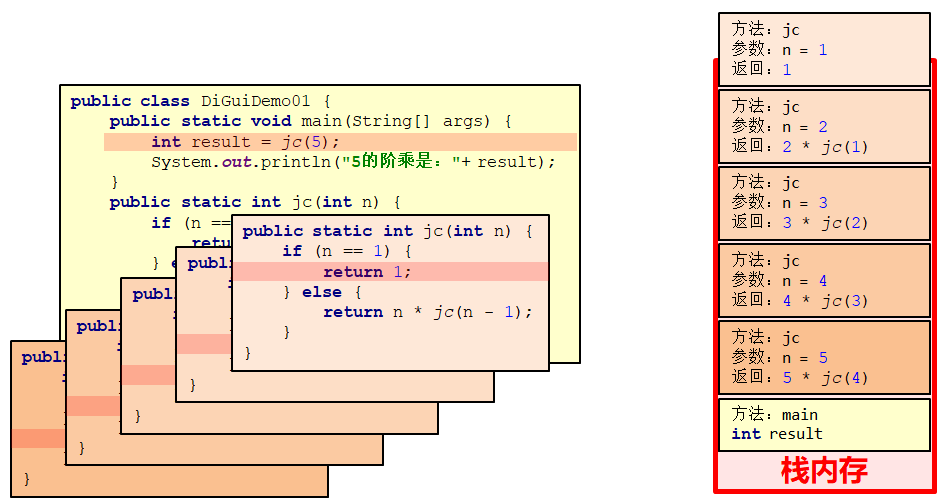## 5.数组的高级操作#

### 5.1 二分查找 (理解)#

• 二分查找概述

查找指定元素在数组中的位置时,以前的方式是通过遍历,逐个获取每个元素,看是否是要查找的元素,这种方式当数组元素较多时,查找的效率很低

二分查找也叫折半查找,每次可以去掉一半的查找范围,从而提高查找的效率

• 需求

在数组{1,2,3,4,5,6,7,8,9,10}中,查找某个元素的位置

• 实现步骤

1. 定义两个变量，表示要查找的范围。默认min = 0 ，max = 最大索引
2. 循环查找，但是min <= max
3. 计算出mid的值
4. 判断mid位置的元素是否为要查找的元素，如果是直接返回对应索引
5. 如果要查找的值在mid的左半边，那么min值不变，max = mid -1.继续下次循环查找
6. 如果要查找的值在mid的右半边，那么max值不变，min = mid + 1.继续下次循环查找
7. 当min > max 时，表示要查找的元素在数组中不存在，返回-1.
• 代码实现

``````public class MyBinarySearchDemo {
public static void main(String[] args) {
int [] arr = {1,2,3,4,5,6,7,8,9,10};
int number = 11;

//1,我现在要干嘛? --- 二分查找
//2.我干这件事情需要什么? --- 数组 元素
//3,我干完了,要不要把结果返回调用者 --- 把索引返回给调用者
int index = binarySearchForIndex(arr,number);
System.out.println(index);
}

private static int binarySearchForIndex(int[] arr, int number) {
//1,定义查找的范围
int min = 0;
int max = arr.length - 1;
//2.循环查找 min <= max
while(min <= max){
//3.计算出中间位置 mid
int mid = (min + max) >> 1;
//mid指向的元素 > number
if(arr[mid] > number){
//表示要查找的元素在左边.
max = mid -1;
}else if(arr[mid] < number){
//mid指向的元素 < number
//表示要查找的元素在右边.
min = mid + 1;
}else{
//mid指向的元素 == number
return mid;
}
}
//如果min大于了max就表示元素不存在,返回-1.
return -1;
}

}
``````
• 注意事项

有一个前提条件，数组内的元素一定要按照大小顺序排列，如果没有大小顺序，是不能使用二分查找法的

### 5.2 冒泡排序 (理解)#

• 冒泡排序概述

一种排序的方式，对要进行排序的数据中相邻的数据进行两两比较，将较大的数据放在后面，依次对所有的数据进行操作，直至所有数据按要求完成排序

如果有n个数据进行排序，总共需要比较n-1次

每一次比较完毕，下一次的比较就会少一个数据参与

• 代码实现

``````public class MyBubbleSortDemo2 {
public static void main(String[] args) {
int[] arr = {3, 5, 2, 1, 4};
//1 2 3 4 5
bubbleSort(arr);
}

private static void bubbleSort(int[] arr) {
//外层循环控制的是次数 比数组的长度少一次.
for (int i = 0; i < arr.length -1; i++) {
//内存循环就是实际循环比较的
//-1 是为了让数组不要越界
//-i 每一轮结束之后,我们就会少比一个数字.
for (int j = 0; j < arr.length - 1 - i; j++) {
if (arr[j] > arr[j + 1]) {
int temp = arr[j];
arr[j] = arr[j + 1];
arr[j + 1] = temp;
}
}
}

printArr(arr);
}

private static void printArr(int[] arr) {
for (int i = 0; i < arr.length; i++) {
System.out.print(arr[i] + " ");
}
System.out.println();
}

}
``````

### 5.3 快速排序 (理解)#

• 快速排序概述

冒泡排序算法中,一次循环结束,就相当于确定了当前的最大值,也能确定最大值在数组中应存入的位置

快速排序算法中,每一次递归时以第一个数为基准数,找到数组中所有比基准数小的.再找到所有比基准数大的.小的全部放左边,大的全部放右边,确定基准数的正确位置

• 核心步骤

1. 从右开始找比基准数小的
2. 从左开始找比基准数大的
3. 交换两个值的位置
4. 红色继续往左找，蓝色继续往右找，直到两个箭头指向同一个索引为止
5. 基准数归位
• 代码实现

``````public class MyQuiteSortDemo2 {
public static void main(String[] args) {
//        1，从右开始找比基准数小的
//        2，从左开始找比基准数大的
//        3，交换两个值的位置
//        4，红色继续往左找，蓝色继续往右找，直到两个箭头指向同一个索引为止
//        5，基准数归位
int[] arr = {6, 1, 2, 7, 9, 3, 4, 5, 10, 8};

quiteSort(arr,0,arr.length-1);

for (int i = 0; i < arr.length; i++) {
System.out.print(arr[i] + " ");
}
}

private static void quiteSort(int[] arr, int left, int right) {
// 递归结束的条件
if(right < left){
return;
}

int left0 = left;
int right0 = right;

//计算出基准数
int baseNumber = arr[left0];

while(left != right){
//        1，从右开始找比基准数小的
while(arr[right] >= baseNumber && right > left){
right--;
}
//        2，从左开始找比基准数大的
while(arr[left] <= baseNumber && right > left){
left++;
}
//        3，交换两个值的位置
int temp = arr[left];
arr[left] = arr[right];
arr[right] = temp;
}
//基准数归位
int temp = arr[left];
arr[left] = arr[left0];
arr[left0] = temp;

// 递归调用自己,将左半部分排好序
quiteSort(arr,left0,left-1);
// 递归调用自己,将右半部分排好序
quiteSort(arr,left +1,right0);

}
}
``````

### 5.4 Arrays (应用)#

• Arrays的常用方法

方法名 说明
public static String toString(int[] a) 返回指定数组的内容的字符串表示形式
public static void sort(int[] a) 按照数字顺序排列指定的数组
public static int binarySearch(int[] a, int key) 利用二分查找返回指定元素的索引
• 示例代码

``````public class MyArraysDemo {
public static void main(String[] args) {
//        public static String toString(int[] a)    返回指定数组的内容的字符串表示形式
//        int [] arr = {3,2,4,6,7};
//        System.out.println(Arrays.toString(arr));

//        public static void sort(int[] a)	  按照数字顺序排列指定的数组
//        int [] arr = {3,2,4,6,7};
//        Arrays.sort(arr);
//        System.out.println(Arrays.toString(arr));

//        public static int binarySearch(int[] a, int key) 利用二分查找返回指定元素的索引
int [] arr = {1,2,3,4,5,6,7,8,9,10};
int index = Arrays.binarySearch(arr, 0);
System.out.println(index);
//1,数组必须有序
//2.如果要查找的元素存在,那么返回的是这个元素实际的索引
//3.如果要查找的元素不存在,那么返回的是 (-插入点-1)
//插入点:如果这个元素在数组中,他应该在哪个索引上.
}
}
``````
• 工具类设计思想

1. 构造方法用 private 修饰
2. 成员用 public static 修饰